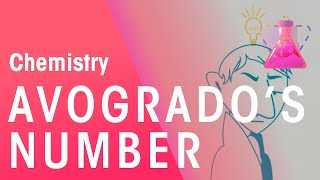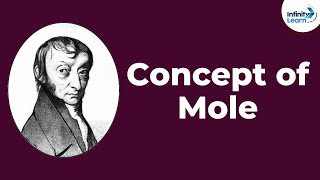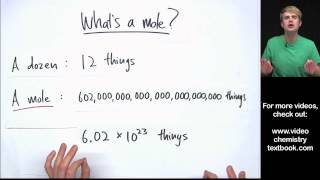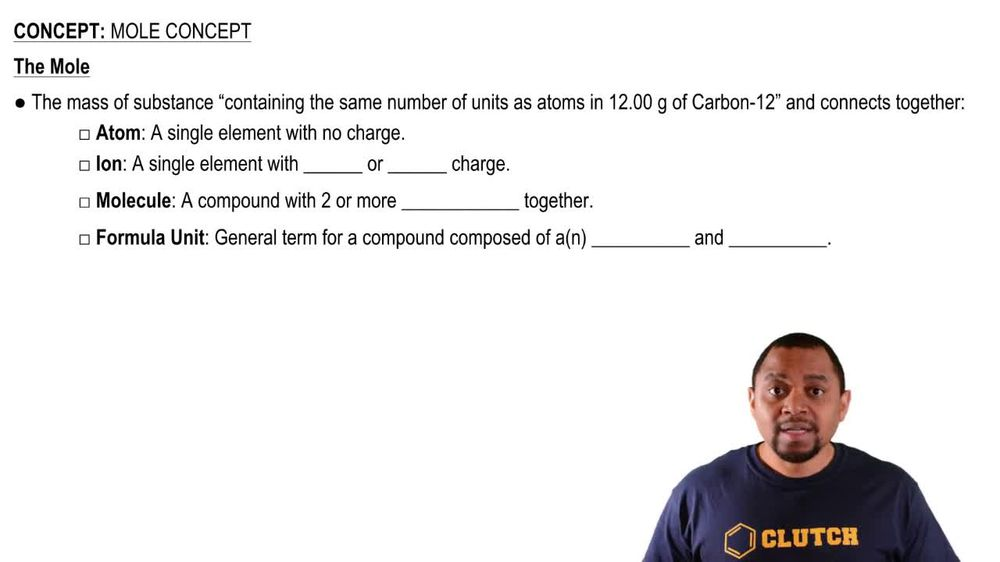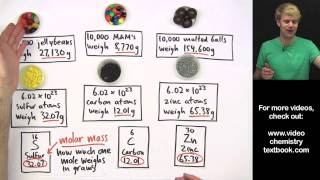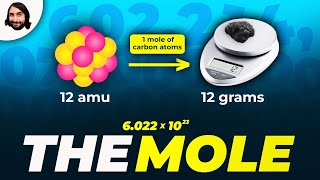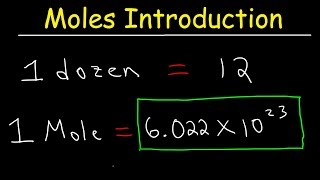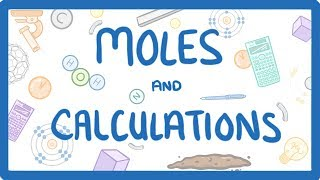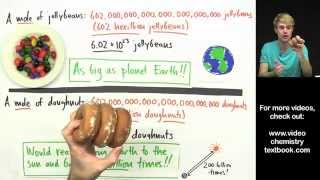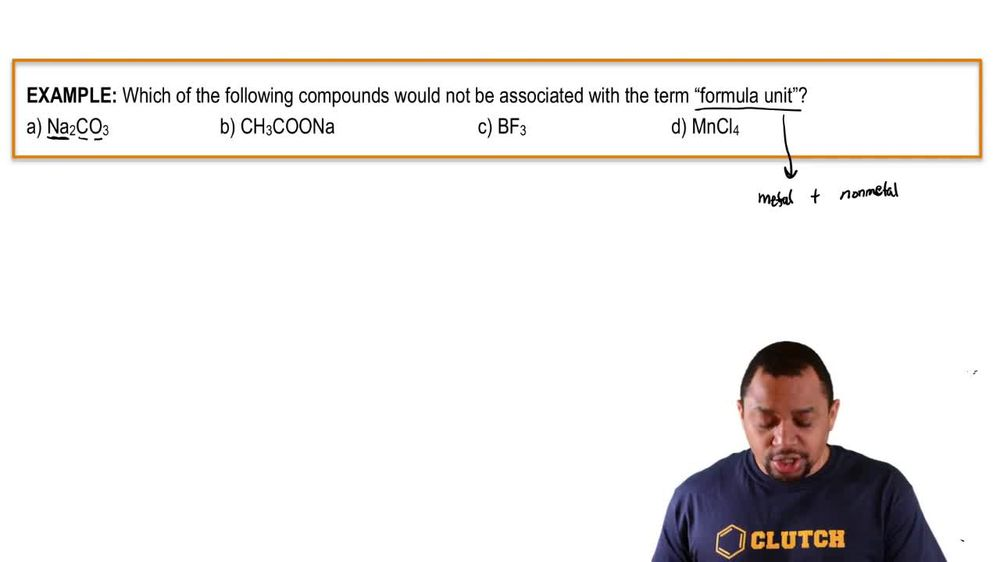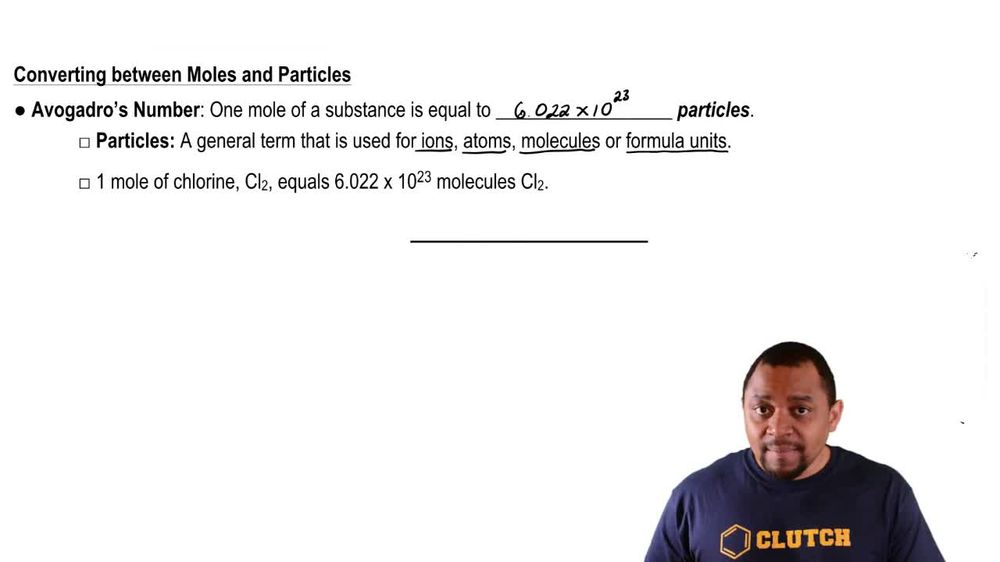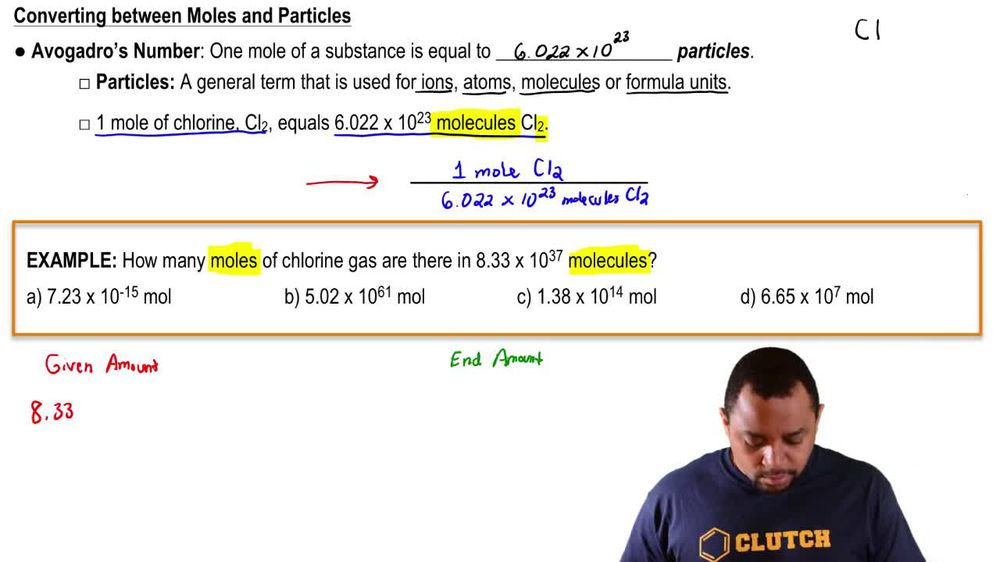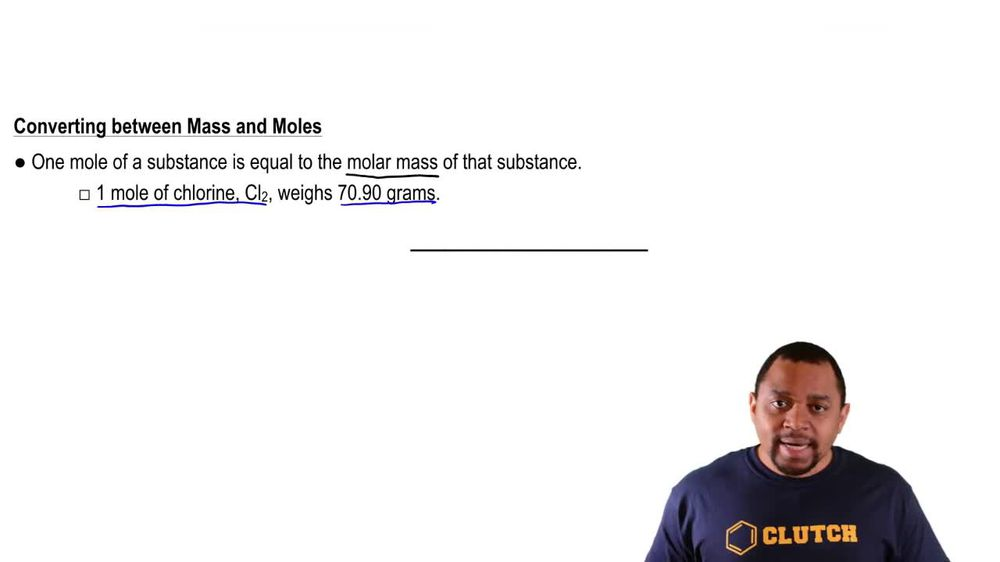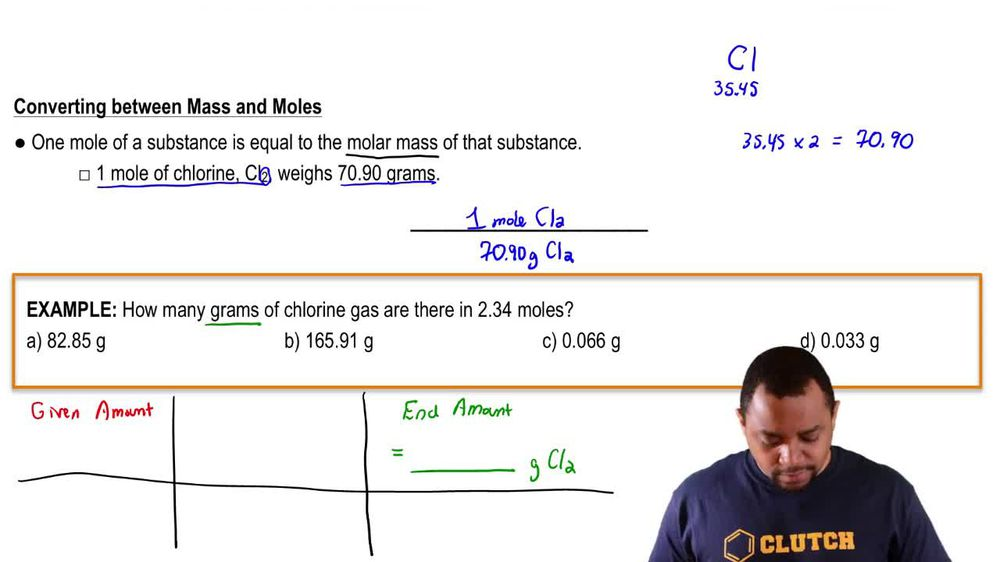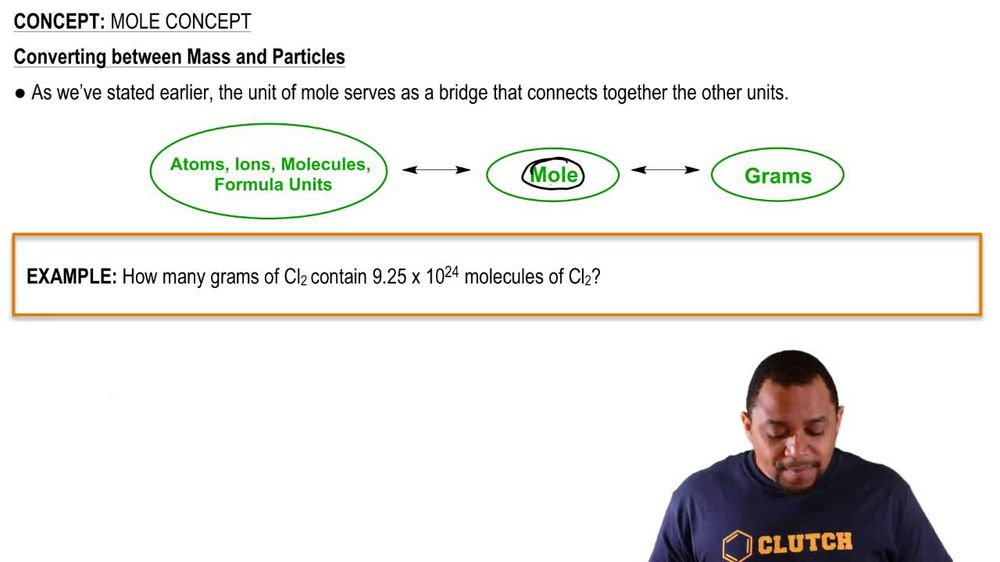Start typing, then use the up and down arrows to select an option from the list.
1. 2. Atoms & Elements2. Mole Concept# Mole Concept Example 3

by Jules Bruno
124 views
3
0
So in this example question, it says, how many grams of chlorine gas are there in 2. moles? All right, so the 2.34 moles represents are given amount, and we have to determine what our end amount will be. What are we trying to get to? So our end amount that we need to find is Gramps. Okay, so here we need to determine the grams off chlorine gas now to go from given amount to end amount. We know we have to utilize a conversion factor, So the conversion factor we have up above is the one that we're going to use. So let's write down are given amount of 2.34 moles of C l two. We need to cancel moles of CL too. So it goes on the bottom. So just like I said earlier, you can invert your conversion factor. You do that to make sure that units cancel out to cancel out these moles in red. I need to put these moles in blue on the bottom and then that one mole of C L two is equal to 70.90 g of c l two So now moles of CL to cancel out and we'll be left with grams of C L two at the end. So when you punch that into your calculator, you should get 65.906 and out of the choices presented be would be the best choice. So just remember, our moles we've seen act as a bridge to connect us to particles earlier, and now we're seeing it connecting us to the mass off chlorine gas.